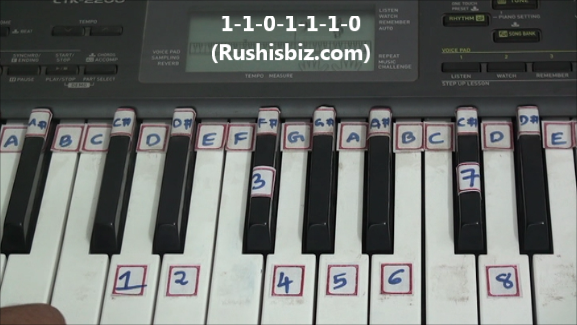HINDI - A B C D E F G H I J K L M N O P Q R S T U V W X Y Z

TAMIL - A B C D E F G H I J K L M N O P Q R S T U V W X Y Z

TELUGU - A B C D E F G H I J K L M N O P Q R S T U V W X Y Z

# “D” Major Scale Positions – Piano Tutorials

Notes: D-1, E-2, F#-3, G-4, A-5, B-6, C#-7, D-8

Since it is a ‘D’ major scale obviously ‘D’ will be first note, how to find the remaining notes ?

The simple formula which is used for any major scale:

One- One- Zero- One-One- One-Zero  (1-1-0-1-1-1-0)

What do you mean by Ones and Zeros ?

One’s (whole step) – If there is a one key between two notes, then the interval of those two notes is One.

Eg: from D to E the interval is “one key”

Zero’s (half step) – If there are no keys or zero keys between two notes, then the interval of those two notes is Zero

Eg: from F# to G the interval is “zero” (Immediate keys)‘D’ Major Scale Positions – Video Tutorials